# Vector and matrix operators. Array addition

## |Vector and matrix operators

The following operators have special meanings for vectors and matrices. Many of these operators are available from the symbol toolbar, but all have corresponding keystrokes. Note that operators which expect vectors always expect column vectors rather than row vectors.

 Operator: Keystroke: addition + cross product Ctrl+8 determinant | division / inner (dot) product * inverse ^-1 magnitude | multiplication * powers of matrix ^ negation - subscript (vector) [ subscript (matrix) [ subtraction - sum elements Ctrl+4 superscript Ctrl+6 transpose Ctrl+1 vectorize Ctrl+-

Keystroke: +

A + B

Adds the elements of A to the corresponding elements of B.

A + x

Adds x to every element in A.

#### Operands: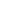A and B are arrays with the same number of rows and columns.The elements of A and B must have the same dimensions.x is any scalar, real or complex.x must have the same dimensions as the elements of A.

## Vector cross product

Keystroke: Ctrl+8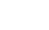Returns the vector cross product of u and v.

#### Operands:Both u and v are three element column vectors.is a vector with the following properties:Its direction is orthogonal to u and v in the direction determined by the right hand rule.Its magnitude is | u || v |sin(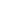) whereis the angle between u and v.

Keystroke: |

| M |

Returns the determinant of a square matrix.

#### Operands:M is a square matrix.

To take the magnitude of each element in a matrix,Place the | M | between the editing lines.Apply the vectorize operator, Ctrl+-.

Although you can use the determinant operator to find numerical determinants, you can also find symbolic determinants.

## Matrix division

Keystroke: /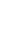Divides every element of A by z.

#### Operands:If A is a matrixz is a nonzero scalar

To divide every element of an m x n matrix A by the corresponding element of another m x n matrix B select the entire expression and press Ctrl+- to insert the vectorize operator.

Keystroke: *Returns the vector inner product (sometimes called a "dot product") of u and v.

#### Operands:Both u and v are vectors having the same number of elements.

The inner product (or dot product) is a scalar formed by multiplying corresponding elements of u and v and summing the results. The inner product has the following properties:=| u || v |cos(q) where q is the angle between u and v.When u and v are orthogonal, their inner product is zero.The inner product of u with itself is simply the square of the length of u.

Keystroke: ^-1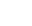This returns the inverse of a matrix. If M does not have an inverse, you'll see an appropriate error message.

#### Operands:M is a square matrix having an inverse.

## Vector magnitude

Keystroke: |

| v |

Returns the magnitude of the vector v. This is the square root of the sum of the squared magnitude of the elements in v.

#### Operands:v is a vector

To take the magnitude of each element in a vector:

1.  Place the | v | between the editing lines.

2.  Apply the vectorize operator, Ctrl+-.

Keystroke: *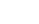Returns the matrix product of A and B.

#### Operands:If A is an m x n matrixB is an n x p matrix

#### Notes:

·  If A is a matrix and x is scalar, then the product of A and x is a matrix in which each element of A has been multiplied by x.

·  To get the term by term product of two equal size arrays A and B, select the entire expression and press Ctrl+- to insert the vectorize operator.

Keystroke: ^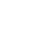Depending on n, this returns one of the following:

### Информация о работе

Тип:
Программы для учёбы
Размер файла:
88 Kb
Скачали:
0